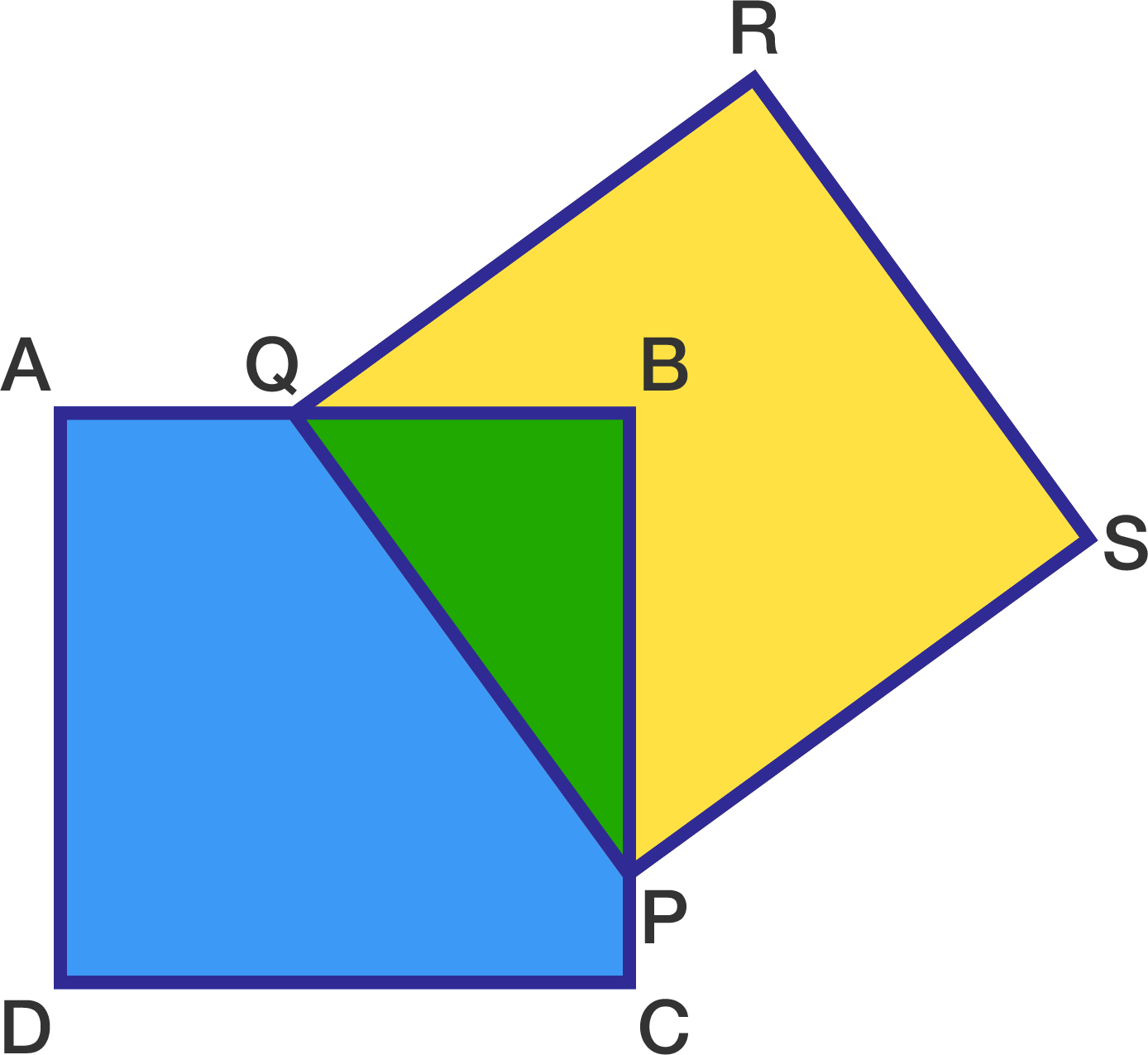# Imagine it

Geometry Level 3Two congruent squares $ABCD$ and $PQRS$ are positioned such that they share a common area defined by $\Delta PQB$. The ratio of the area of $\Delta PQB$ to the area of polygon $AQRSPCD$ is $\dfrac{3}{22}$.

If the side length of both squares is $s$, then the perimeter of polygon $AQRSPCD$ is $\dfrac{m}{n}s$, where $m$ and $n$ are coprime positive integers. Find $m+n$.

×